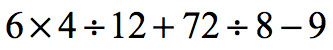PEMDAS 1
PEMDAS 2
PEMDAS 3
PEMDAS 4
PEMDAS 5
100

What does the P stand for?

Parentheses

100

What does the E stand for?

Exponent

100

What does the M stand for?

Multiplication

100

What does the D stand for?

Division

100

What does the A stand for?

200

What does the S stand for?

Subtraction

200

What do I do First in PEMDAS?

Parentheses

200

What do I do Last in PEMDAS

Subtraction

200

When do I have to apply the Left to Right rule?

When the problem has only MD or AS.

200

What rule should you use when you see only Multiplying and Dividing in the problem?

Left to Right rule

300

What is the answer?

10 ÷ 2 ✖  3

15

300

What is the answer?

(9- 32) + 5

5

300

What is the answer?

8 - 7 + 5 ✖ 2

11

300

What is the answer?

42 ÷  2  -  6 ✖ 1

2

300

What is the answer?

11+22+33-44

22

400

What is the answer?

34+56-47+120-56

107

400

What is the name of the movie from the character?Casper

400

What is the character from the photo?Elmo

400

What is the answer? 42+69+96-24

183

400

What is the answer?

56x12-457+64

279

500

What is the answer?

(2x3)-7- 18 ÷ 6

-4

500

What does PEMDAS stand for?

What is Parentheses, Exponents, Multiplication, Division, Addition, and Subtraction

500

What is the answer? (5x6)+67+34-3

128

500

What is the answer?

(12x56)+45-12

705

500

What is the answer?2

Click to zoom